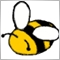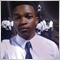# Margin calculation modes490

Since the EA i'm writing will be used only on forex pairs, should I check the SymbolInfoInteger(SYMBOL_TRADE_CALC_MODE) ?
1. is this SymbolInfoInteger(SYMBOL_TRADE_CALC_MODE), a reliable checkup to be sure if EA is activated on a forex pair (and not on OIL and other stuff) ?

and I have added the following (limiting the max leverage usage) to my code :

```// FIN_LOT calculated here.... (is position volume)
// ACC_LEVERAGE : max leverage allowed by account (e.g 100)
// LEVERAGE_CAP based on user input (e.g : 33)
// bla bla bla

double REQ_MARGIN_1_LOT = 0; // required margin for 1 lot

else OrderCalcMargin(ORDER_TYPE_SELL, _Symbol, 1, CurrPrice.bid, REQ_MARGIN_1_LOT);

// checking failures... and then :

if((FIN_LOT*REQ_MARGIN_1_LOT*ACC_LEVERAGE/FREE_MARGIN > LEVERAGE_CAP)
FIN_LOT = (FREE_MARGIN*LEVERAGE_CAP)/(REQ_MARGIN_1_LOT*ACC_LEVERAGE);```
Now, it does the job, but

2. is this calculation limited to SYMBOL_CALC_MODE_FOREX only ? (is this the variable I should check before using the above code ?)
and, if someone knows of a broker with SYMBOL_CALC_MODE_FOREX_NO_LEVERAGE mode, which i can do some tests on its demo accounts, it will be great.

thanks.428

Code2219 or 2319:

Since the EA i'm writing will be used only on forex pairs, should I check the SymbolInfoInteger(SYMBOL_TRADE_CALC_MODE) ?
1. is this SymbolInfoInteger(SYMBOL_TRADE_CALC_MODE), a reliable checkup to be sure if EA is activated on a forex pair (and not on OIL and other stuff) ?

and I have added the following (limiting the max leverage usage) to my code :

Now, it does the job, but

2. is this calculation limited to SYMBOL_CALC_MODE_FOREX only ? (is this the variable I should check before using the above code ?)
and, if someone knows of a broker with SYMBOL_CALC_MODE_FOREX_NO_LEVERAGE mode, which i can do some tests on its demo accounts, it will be great.

thanks.

An answer to this coulda helped.